# Tag Archives: Lp

[an updated version is now online here]

After my series of post on classification algorithms, it’s time to get back to R codes, this time for quantile regression. Yes, I still want to get a better understanding of optimization routines, in R. Before looking at the quantile regression, let us compute the median, or the quantile, from a sample.

## Median

Consider a sample $\{y_1,\cdots,y_n\}$. To compute the median, solve$$\min_\mu \left\lbrace\sum_{i=1}^n|y_i-\mu|\right\rbrace$$which can be solved using linear programming techniques. More precisely, this problem is equivalent to$$\min_{\mu,\mathbf{a},\mathbf{b}}\left\lbrace\sum_{i=1}^na_i+b_i\right\rbrace$$with $a_i,b_i\geq 0$ and $y_i-\mu=a_i-b_i$, $\forall i=1,\cdots,n$.
To illustrate, consider a sample from a lognormal distribution,

n = 101 set.seed(1) y = rlnorm(n) median(y)  1.077415

For the optimization problem, use the matrix form, with $3n$ constraints, and $2n+1$ parameters,

So far so good…

## Quantile Regression (simple)

Consider the following dataset, with rents of flat, in a major German city, as function of the surface, the year of construction, etc.

base=read.table("http://freakonometrics.free.fr/rent98_00.txt",header=TRUE)

The linear program for the quantile regression is now$$\min_{\mu,\mathbf{a},\mathbf{b}}\left\lbrace\sum_{i=1}^n\tau a_i+(1-\tau)b_i\right\rbrace$$with $a_i,b_i\geq 0$ and $$y_i-[\beta_0^\tau+\beta_1^\tau x_i]=a_i-b_i$$$\forall i=1,\cdots,n$. So use here

require(lpSolve) tau = .3 n=nrow(base) X = cbind( 1, base$area) y = base$rent_euro A1 = cbind(diag(2*n), 0,0) A2 = cbind(diag(n), -diag(n), X) r = lp("min", c(rep(tau,n), rep(1-tau,n),0,0), rbind(A1, A2), c(rep("&gt;=", 2*n), rep("=", n)), c(rep(0,2*n), y)) tail(r$solution,2)  148.946864 3.289674 Of course, we can use R function to fit that model library(quantreg) rq(rent_euro~area, tau=tau, data=base) Coefficients: (Intercept) area 148.946864 3.289674 Here again, it seems to work quite well. We can use a different probability level, of course, and get a plot plot(base$area,base$rent_euro,xlab=expression(paste("surface (",m^2,")")), ylab="rent (euros/month)",col=rgb(0,0,1,.4),cex=.5) sf=0:250 yr=r$solution[2*n+1]+r$solution[2*n+2]*sf lines(sf,yr,lwd=2,col="blue") tau = .9 r = lp("min", c(rep(tau,n), rep(1-tau,n),0,0), rbind(A1, A2), c(rep("&gt;=", 2*n), rep("=", n)), c(rep(0,2*n), y)) tail(r$solution,2)  121.815505 7.865536 yr=r$solution[2*n+1]+r$solution[2*n+2]*sf lines(sf,yr,lwd=2,col="blue")## Quantile Regression (multiple)

Now that we understand how to run the optimization program with one covariate, why not try with two ? For instance, let us see if we can explain the rent of a flat as a (linear) function of the surface and the age of the building.

require(lpSolve) tau = .3 n=nrow(base) X = cbind( 1, base$area, base$yearc ) y = base$rent_euro A1 = cbind(diag(2*n), 0,0,0) A2 = cbind(diag(n), -diag(n), X) r = lp("min", c(rep(tau,n), rep(1-tau,n),0,0,0), rbind(A1, A2), c(rep("&gt;=", 2*n), rep("=", n)), c(rep(0,2*n), y)) tail(r$solution,3)  0.000000 3.257562 0.077501

Unfortunately, this time, it is not working well…

library(quantreg) rq(rent_euro~area+yearc, tau=tau, data=base) Coefficients: (Intercept) area yearc -5542.503252 3.978135 2.887234

Results are quite different. And actually, another technique can confirm the later (IRLS – Iteratively Reweighted Least Squares)

eps = residuals(lm(rent_euro~area+yearc, data=base)) for(s in 1:500){ reg = lm(rent_euro~area+yearc, data=base, weights=(tau*(eps&gt;0)+(1-tau)*(eps&lt;0))/abs(eps)) eps = residuals(reg) } reg$coefficients (Intercept) area yearc -5484.443043 3.955134 2.857943 I could not figure out what went wrong with the linear program. Not only coefficients are very different, but also predictions… yr = r$solution[2*n+1]+r$solution[2*n+2]*base$area+r$solution[2*n+3]*base$yearc plot(predict(reg),yr) abline(a=0,b=1,lty=2,col="red")It’s now time to investigate….

# Convergences de variables aléatoiresLe schéma – ou diagramme – des relations entre les modes convergences ressemble à ce qui est présenté sur la droite. C’est grosso modo ce que j’ai dit en cours (sans le représenter explicitement).

Je ne vais pas démontrer ces implications car ce sont des théorèmes (qui peuvent donc se trouver dans n’importe quel textbook de probabilité).

En revanche, un étudiant m’avait demandé si les réciproques étaient vraies. Comme toujours en maths, si les réciproques étaient toujours vraies, on le mettrait. Donc ici les réciproques sont généralement fausses. On peut toutefois se demander quand ces réciproques peuvent être vraies…et quand elles sont fausses.

• de la convergence en proba à la convergence en moyenne

Commençons par le plus simple (à mon avis). Ilexiste un cas particulier où cette réciproque est vraie: le cas de variables aléatoires bornées. Autrement dit, si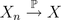et que l’on a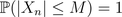pour tout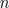, alors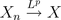pour tout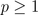.
Bon, en fait la condition est un peu trop forte, et il suffit que la suite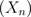soit uniformément intégrable.
Maintenant, il existe aussi des cas où cette réciproque sera fausse (et on se doute qu’en construisant une suite non bornée, on devrait y arriver). Par exemple, supposons que pour tout,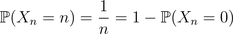On peut montrer que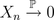, car en effet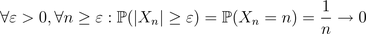Toutefois, certes la suite converge dans L1, mais vers une limite différente, étant donné que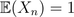pour tout. De plus,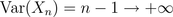et donc la suite ne converge pas dans L2.

• de la convergence en loi à la convergence en probaUn exemple simple ici: siconverge en loi vers une constante, alors on aura convergence en probabilité (vers la même constante).
Maintenant la réciproque est relativement simple, l’idée étant quen’implique en rien, au sens où, pour tout. Supposons maintenant que l’on ait à la foiset, où on va supposer que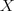est une loi symétrique. La symétrie signifie que. Notons que l’on a alors forcément. Supposons que la réciproque soit toujours vraie, alors on devrait avoir, et donc on aurait que, ce qui n’arrive que siest la constante.

• de la convergence en proba vers la convergence presque sûre

Je ne vais pas m’étendre sur la construction d’exemples pour lesquels la réciproque est fausse (formellement, il suffit de changer d’espace de probabilité sous-jacent, je peux renvoyer vers les notes de cours de Philippe Briand (ici) et Hélène m’a suggéré également les exercices en ligne ). Par contre, il existe des cas importants où la réciproque est vraie.
Considérons une suite de variables indépendantes et identiquement distribuées. Posons alors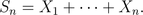On peut alors montrer que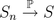si et seulement si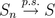(ça doit être du Borel-Cantelli).

• de la convergence en loi à la convergence presque sûre

Je ne vais pas non plus m’étendre davantage, mais on a un résultat qui ressemble à ce que je disais auparavant. En fait, il existe un théorème (dit de Skorohod) qui permet de dire que si on a une convergence en loi, alors on peut construite une suite de variables aléatoires qui
convergera presque sûrement. Formellement on a que si, alors il existe une suite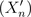et une variable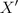, telles que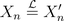et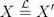telles que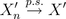. Mais de manière générale, si la suite n’est pas construite comme il faut (paren quelques sortes) alors il est assez rare d’avoir la réciproque.